# LeetCode周赛324，质量不错的福利场，推荐萌新一刷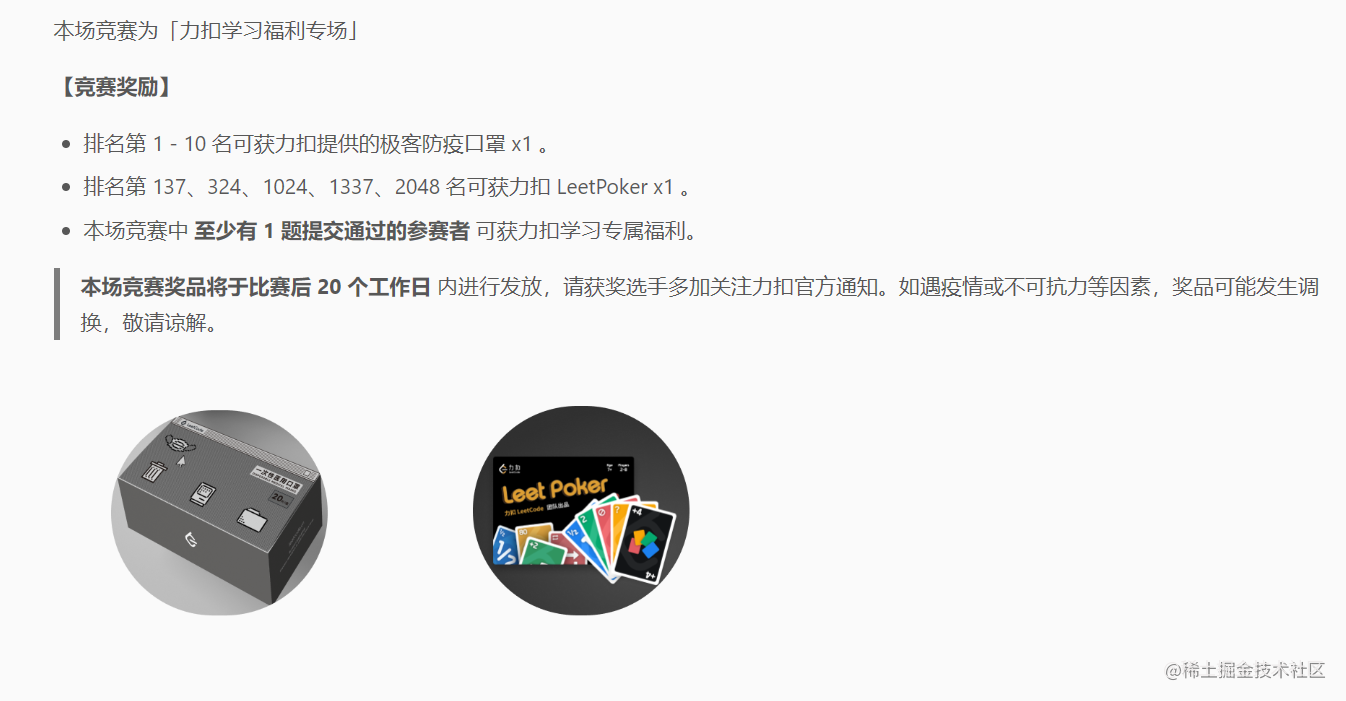## 统计相似字符串对的数目

• 例如，"abca""cba" 相似，因为它们都由字符 'a''b''c' 组成。
• 然而，"abacba""bcfd" 不相似，因为它们不是相同字符组成的。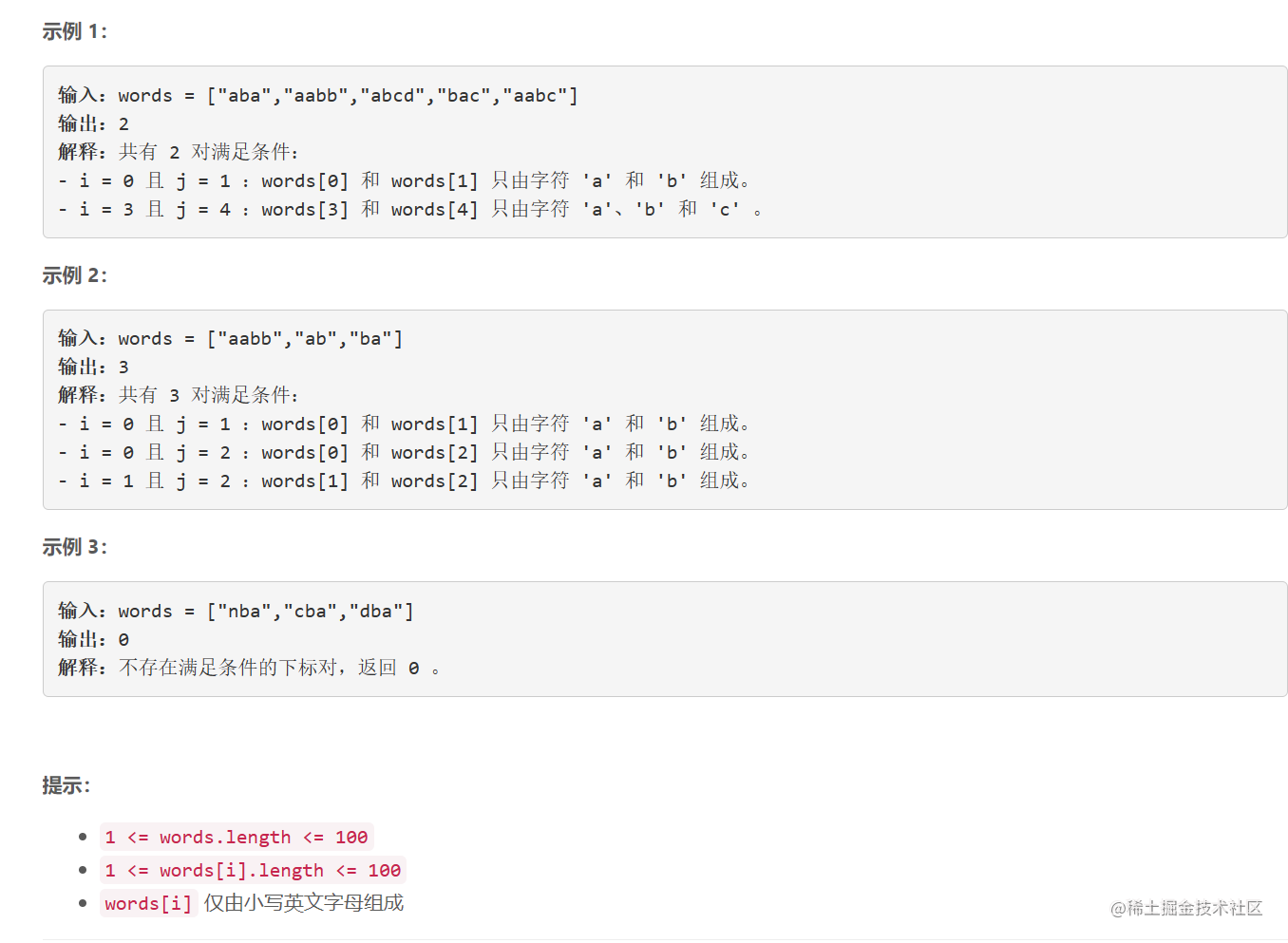### 题解

class Solution {
public:
int similarPairs(vector<string>& words) {
map<int, int> mp;
for (auto & w: words) {
// 将字符串构成转化成整数
int tmp = 0;
for (auto c : w) {
tmp |= (1 << (c - 'a'));
}
mp[tmp]++;
}
int ret = 0;
for (auto it : mp) {
if (it.second > 1) {
// 假设某一个构成出现x次，那么对应的字符串对数量为C(x, 2)，即x(x-1)/2
ret += (it.second * (it.second - 1)) / 2;
}
}
return ret;
}
};

## 使用质因数之和替换后可以取到的最小值

• 注意，如果 n 能够被某个质因数多次整除，则在求和时，应当包含这个质因数同样次数。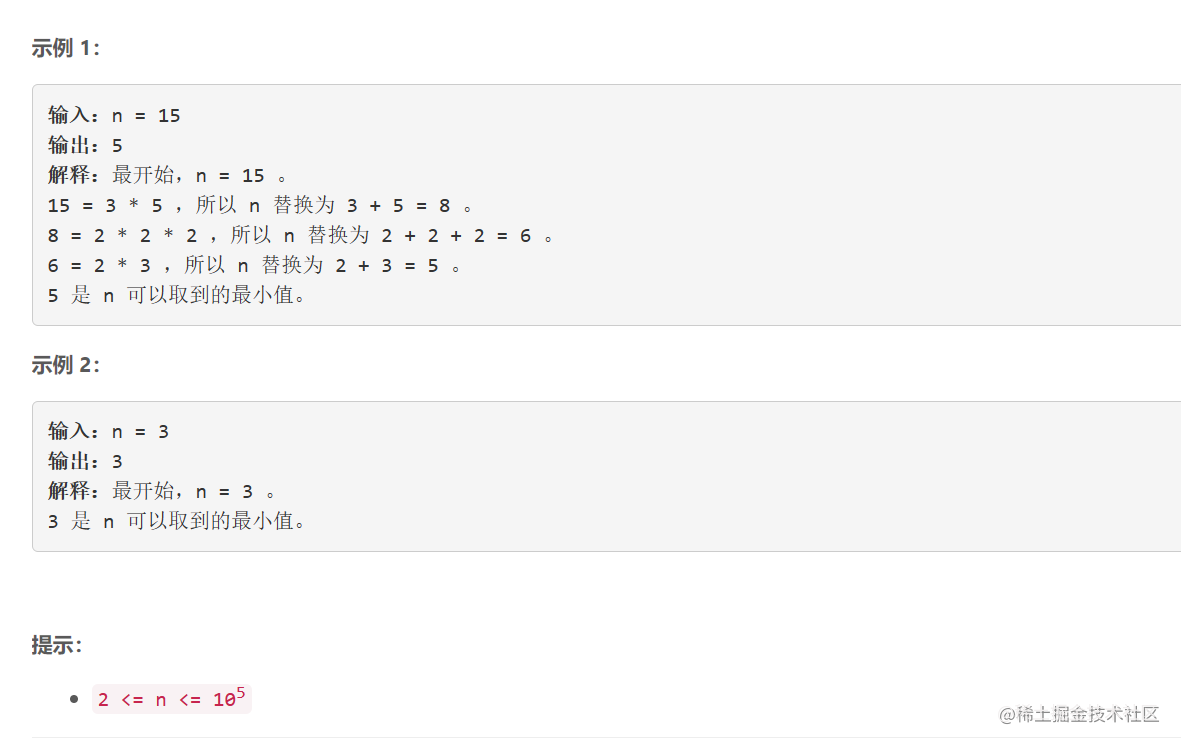### 题解

class Solution {
public:
int smallestValue(int n) {
vector<bool> is_prime(100050, 1);
vector<int> prime;

// 筛法，筛选出1e5范围内的所有素数
const int N = 100001;
for (int i = 2; i < N; i++) {
if (is_prime[i]) {
prime.push_back(i);
for (int j = i * 2; j < N; j+=i) {
is_prime[j] = 0;
}
}
}

int ret = n;
int tmp = 0;
// n不再发生变化时退出
while (true) {
tmp = 0;
// 记录之前的n
int cur = n;
while (n > 1) {
for (auto x : prime) {
while (n % x == 0) {
n = n / x;
tmp += x;
}
}
}
// 如果变化前后一样，则break
if (cur == tmp) break;
n = tmp;
ret = min(ret, tmp);
}
return ret;
}
};

## 添加边使所有节点度数都为偶数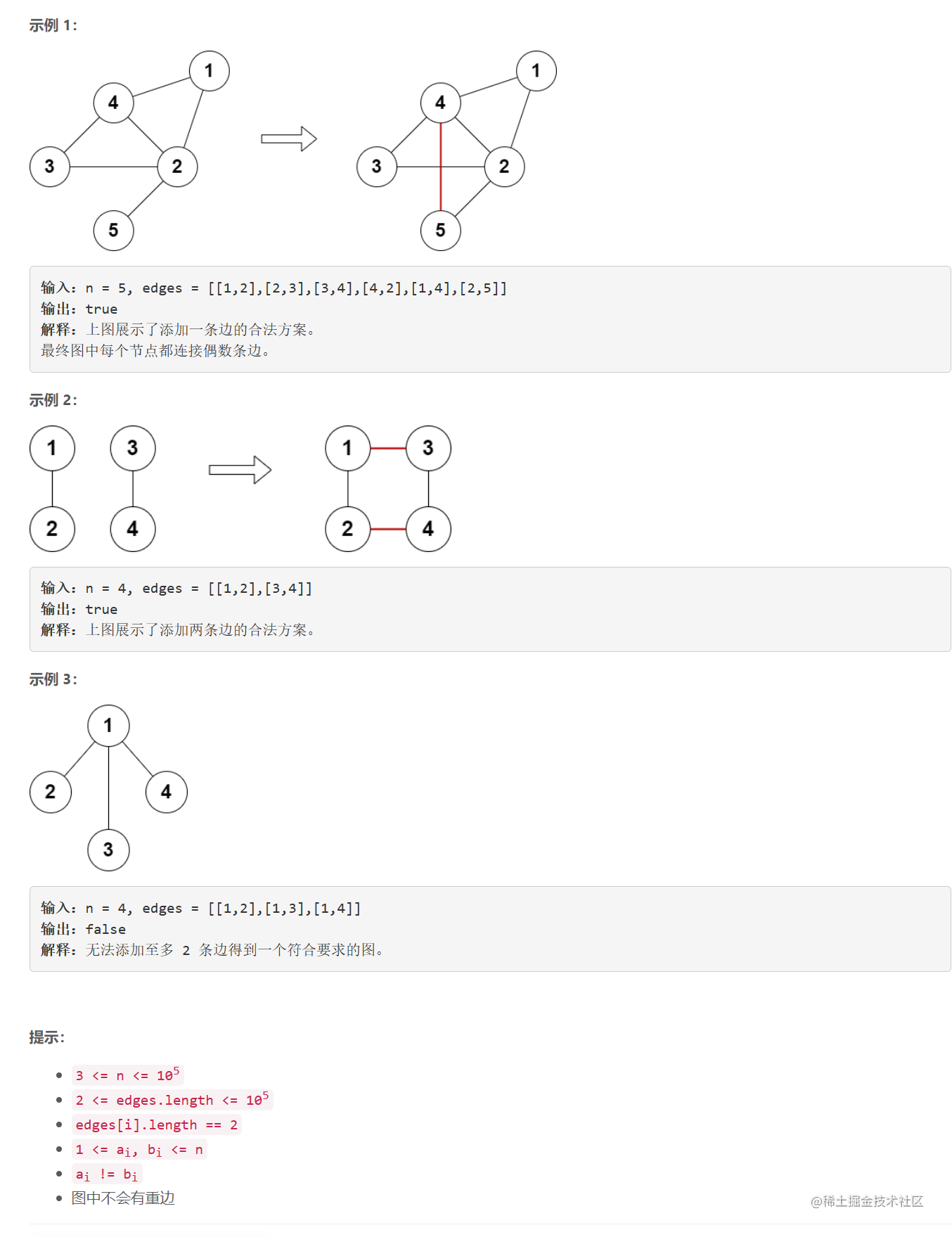### 题解

class Solution {
public:
bool isPossible(int n, vector<vector<int>>& edges) {
// 邻接表建图
vector<int> graph[n+1];
for (auto &vt: edges) {
int u = vt, v = vt;
graph[u].push_back(v);
graph[v].push_back(u);
}

// 根据点的度数划分奇偶
// 邻接表建图之后可以直接得到每个点的度数
vector<int> even, odd;
for (int i = 1; i <= n; i++) {
if (graph[i].size() % 2) {
odd.push_back(i);
}else {
even.push_back(i);
}
}

if (odd.size() == 0) return true;
if (odd.size() == 2) {
int u = odd, v = odd;
// 如果u和v不相连，返回true
bool flag = true;
for (auto x: graph[u]) if (x == v) flag = false;
if (flag) return true;

// 维护u和v所有连接的点
set<int> nodes;
for (auto x: graph[u]) nodes.insert(x);
for (auto x: graph[v]) nodes.insert(x);

nodes.insert(u);
nodes.insert(v);

// 如果存在一个点和u、v都不相连，则可以让u、v都连上
return nodes.size() < n;
}
if (odd.size() == 4) {
auto check_comb = [&](int u1, int u2, int v1, int v2) -> bool {
u1 = odd[u1], u2 = odd[u2];
v1 = odd[v1], v2 = odd[v2];
// 判断u1-u2, v1-v2是否相连
bool flag = true;
for (auto x: graph[u1]) if (x == u2) return false;
for (auto x: graph[v1]) if (x == v2) return false;
return true;
};
return check_comb(0, 1, 2, 3) || check_comb(0, 2, 1, 3) || check_comb(0, 3, 1, 2);
}
return false;
}
};

## 查询树中环的长度

• 左子节点的编号为 2 * val
• 右子节点的编号为 2 * val + 1

1. 在节点编号为 aibi 之间添加一条边。
2. 求出图中环的长度。
3. 删除节点编号为 aibi 之间新添加的边。

• 是开始和结束于同一节点的一条路径，路径中每条边都只会被访问一次。
• 环的长度是环中边的数目。
• 在树中添加额外的边后，两个点之间可能会有多条边。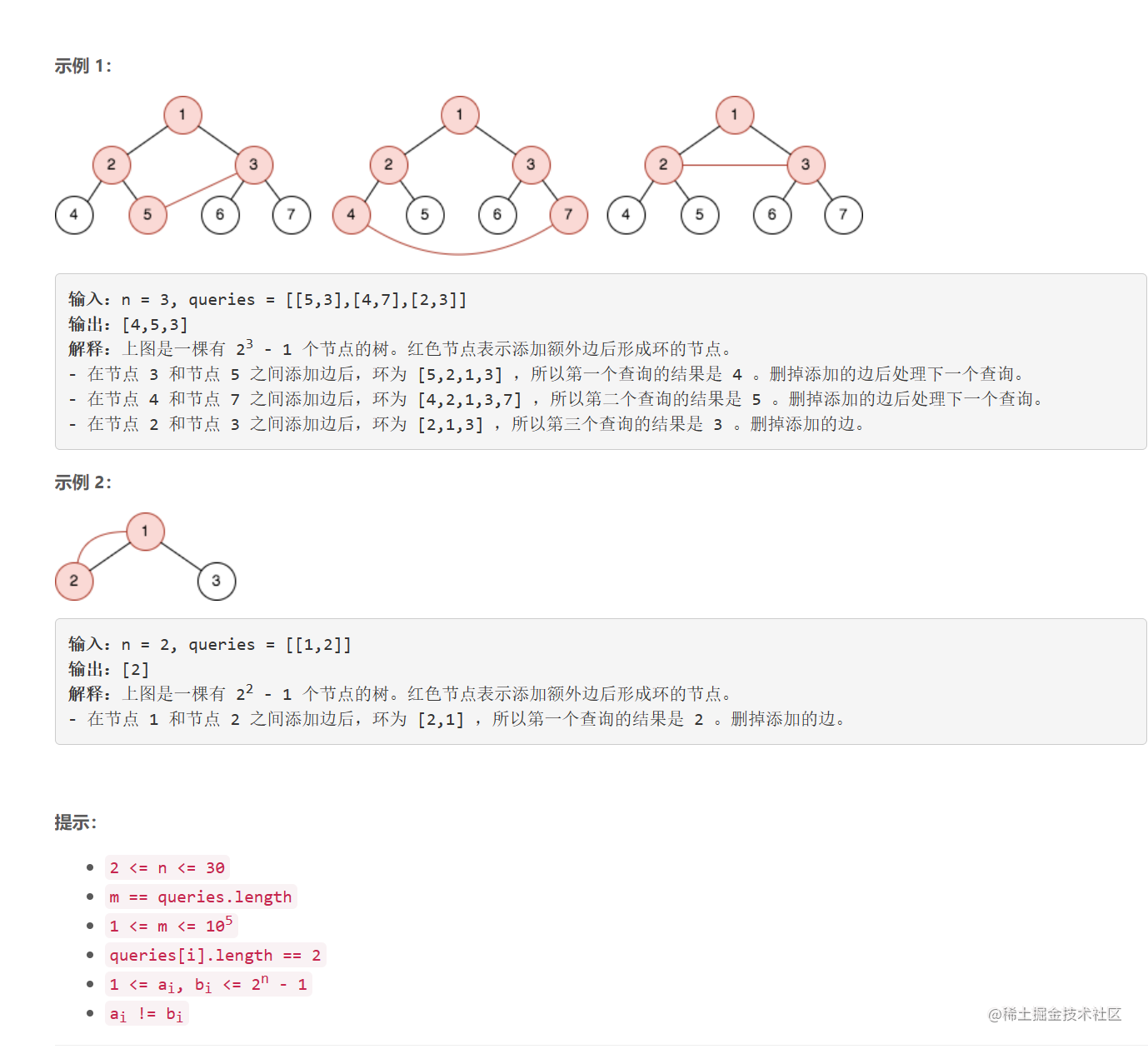### 题解

class Solution {
public:
vector<int> cycleLengthQueries(int n, vector<vector<int>>& queries) {
vector<int> ret;

for (auto &q: queries) {
int u = q, v = q;
// 分别记录u、v的路径长度
int l = 0, r = 0;
while (u != v) {
if (u > v) {
u = u >> 1;
l++;
}else {
v = v >> 1;
r++;
}
}
// 答案为l+r+1
ret.push_back(l+r+1);
}
return ret;
}
};